# 03 封装和解构

1,封装和解构

1.1 基本概念

t1 = 1, 2
print(type(t1)) # 什么类型
t2 = (1, 2)
print(type(t2))

Python等式右侧出现逗号分隔的多值的时候，就会将这几个值封装到元组中。这种操作称为封装 packing。

x, y = (1, 2)
print(x) # 1
print(y) # 2

Python中等式右侧是一个容器类型，左侧是逗号分隔的多个标识符，将右侧容器中数据的一个个和左侧 标识符一一对应。这种操作称为解构unpacking。

# 交换数据
x = 4
y = 5
t = x
x = y
y = t
# 封装和解构，交换
x = 10
y = 11
x, y = y, x

1.2 简单结构

# 左右个数相同
a,b = 1,2
a,b = (1,2)
a,b = [1,2]
a,b = [10,20]
a,b = {10,20} # 非线性结构
a,b = {'a':10,'b':20} # 非线性结构也可以解构
[a,b] = (1,2)
[a,b] = 10,20
(a,b) = {30,40}

1.3 剩余变量解构

a, *rest, b = [1, 2, 3, 4, 5]
print(a, b)
print(type(rest), rest) # <class 'list'> [2, 3, 4]

a, *rest = [1, 2, 3, 4, 5]
print(a, rest)
*rest, b = [1, 2, 3, 4, 5]
print(rest, b)
*rest = [1, 2, 3, 4, 5]
print(rest) # 内容是什么？
a, *r1, *r2, b = [1, 2, 3, 4, 5] # ？
a, *_, b = [1, 2, 3, 4, 5]
print(_) # 在IPython中实验，_是最后一个输出值，这里将把它覆盖
_, *b, _ = [1, 2, 3]
print(_) # 第一个_是什么
print(b) # 是什么
print(_) # 第二个_是什么

_是合法的标识符，这里它没有什么可读性，它在这里的作用就是表示不关心这个变量的值，我不想 要。有人把它称作 丢弃(Throwaway)变量。

2, 集合Set

Python中，它是可变的、无序的、不重复的元素的集合

2.1 初始化

set() -> new empty set objectset(iterable) -> new set object
s1 = set()
s2 = set(range(5))
s3 = set([1, 2, 3])
s4 = set('abcdabcd')
s5 = {} # 这是什么？
s6 = {1, 2, 3}
s7 = {1, (1,)}
s8 = {1, (1,), } # ？

2.2 元素性质

2.3 增加

add(elem)

s = set()
s.add(1)
s.update((1,2,3), [2,3,4])

2.4 删除

remove(elem)

s = set(range(10))
s.remove(0)
#s.remove(11) # KeyError为什么 s.discard(11)
s.pop()
s.clear()

2.5 修改

2.6 索引

2.7 遍历

2.8 成员运算符in

print(10 in [1, 2, 3])
print(10 in {1, 2, 3})

2.8.1 IPython魔术方法

IPython内置的特殊方法，使用%百分号开头的

% 开头是line magic%% 开头是 cell magic，notebook的cell
%timeit statement
-n 一个循环loop执行语句多少次
-r 循环执行多少次loop，取最好的结果
%%timeit setup_code
* code.....
# 下面写一行，列表每次都要创建，这样写不好
%timeit (-1 in list(range(100)))
# 下面写在一个cell中，写在setup中，列表创建一次
%%timeit l=list(range(1000000))
-1 in l

2.8.2 set和线性结构比较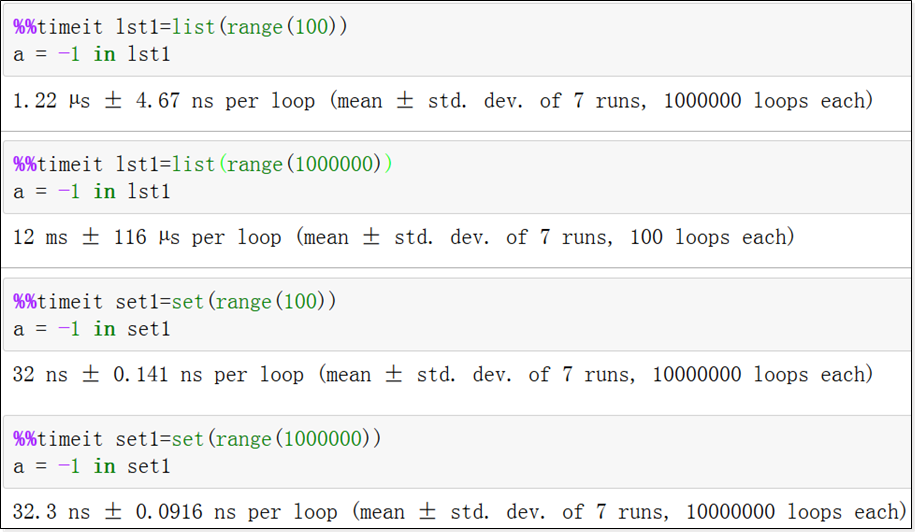2.9 可哈希

set元素必须是可hash的。

2.10 集合概念

A是B的子集，且A不等于B，A就是B的真子集，B是A的真超集并集：多个集合合并的结果交集：多个集合的公共部分差集：集合中除去和其他集合公共部分

2.11 并集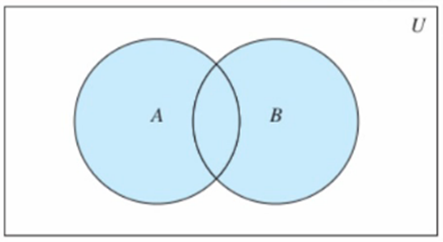union(*others) 返回和多个集合合并后的新的集合| 运算符重载，等同unionupdate(*others) 和多个集合合并，就地修改|= 等同update

2.12 交集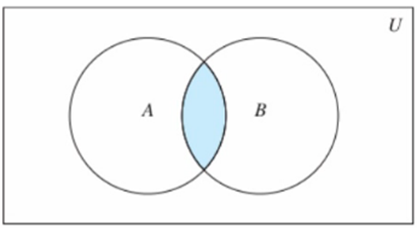intersection(*others) 返回和多个集合的交集& 等同intersectionintersection_update(*others) 获取和多个集合的交集，并就地修改 - &= 等同intersection_update

2.13 差集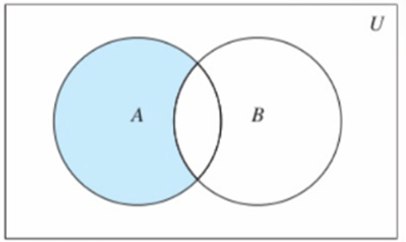difference(*others) 返回和多个集合的差集等同differencedifference_update(*others) 获取和多个集合的差集并就地修改-= 等同difference_update

2.14 对称差集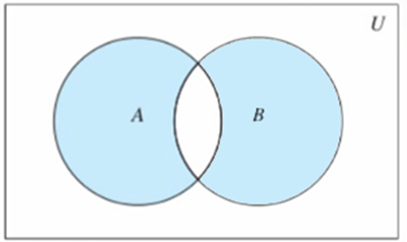symmetric_differece(other) 返回和另一个集合的对称差集^ 等同symmetric_differecesymmetric_differece_update(other) 获取和另一个集合的对称差集并就地修改^= 等同symmetric_differece_update

2.15 其他集合运算

issubset(other)、<= 判断当前集合是否是另一个集合的子集set1 < set2 判断set1是否是set2的真子集issuperset(other)、>= 判断当前集合是否是other的超集set1 > set2 判断set1是否是set2的真超集isdisjoint(other) 当前集合和另一个集合没有交集，没有交集，返回True

3,字典Dict

Dict即Dictionary，也称为mapping。 Python中，字典由任意个元素构成的集合，每一个元素称为Item，也称为Entry。这个Item是由(key, value)组成的二元组。

3.1 初始化

dict(**kwargs) 使用name=value对初始化一个字典dict(iterable, kwarg) 使用可迭代对象和name=value对构造字典，不过可迭代对象的元素 必须是一个二元结构dict(mapping, **kwarg) 使用一个字典构建另一个字典

d1 = {}
d2 = dict()
d3 = dict(a=100, b=200)
d4 = dict(d3) # 构造另外一个字典
d5 = dict(d4, a=300, c=400)
d6 = dict([('a', 100), ['b', 200], (1, 'abc')], b=300, c=400)
# 类方法dict.fromkeys(iterable, value)
d = dict.fromkeys(range(5))
d = dict.fromkeys(range(5), 0)

3.2 元素访问

d[key]

3.3 新增和修改

d[key] = value

d = {}
d['a'] = 1
d.update(red=1)
d.update(['red', 2]
d.update({'red':3})

3.4 删除

pop(key[, default])
key存在，移除它，并返回它的valuekey不存在，返回给定的defaultdefault未设置，key不存在则抛出KeyError异常popitem()

3.5 遍历

1、遍历Key

for k in d:
print(k)
for k in d.keys():
print(k)

2、遍历Value

for v in d.values():
print(v)
for k in d.keys():
print(d[k])
print(d.get(k))

3、遍历Item

for item in d.items():
print(item)
print(item, item)

for k,v in d.items():
print(k, v)
for k,_ in d.items():
print(k)
for _,v in d.items():
print(v)

Python3中，keys、values、items方法返回一个类似一个生成器的可迭代对象

Dictionary view对象，可以使用len()、iter()、in操作字典的entry的动态的视图，字典变化，视图将反映出这些变化keys返回一个类set对象，也就是可以看做一个set集合。如果values都可以hash，那么items也可 以看做是类set对象

Python2中，上面的方法会返回一个新的列表，立即占据新的内存空间。所以Python2建议使用 iterkeys、itervalues、iteritems版本，返回一个迭代器，而不是返回一个copy

3.6 遍历与删除

# 错误的做法
d = dict(a=1, b=2, c=3)
for k,v in d.items():
print(d.pop(k))

while len(d):
print(d.popitem())

while d:
print(d.popitem())

# for 循环正确删除
d = dict(a=1, b=2, c=3)
keys = []
for k,v in d.items():
keys.append(k)
for k in keys:
d.pop(k)

3.7 key

set的元素可以就是看做key，set可以看做dict的简化版hashable 可哈希才可以作为key，可以使用hash()测试使用key访问，就如同列表使用index访问一样，时间复杂度都是O(1)，这也是最好的访问元素的 方式
d = {
1 : 0,
2.0 : 3,
"abc" : None,
('hello', 'world', 'python') : "string",
b'abc' : '135'
}

3.8 有序性

# 3.5如下
C:PythonPython353>python
Python 3.5.3 (v3.5.3:1880cb95a742, Jan 16 2017, 16:02:32) [MSC v.1900 64 bit (AMD64)] on win32
Type "help", "copyright", "credits" or "license" for more information.
>>> d = {'a':300, 'b':200, 'c':100, 'd':50}
>>> d
{'c': 100, 'a': 300, 'b': 200, 'd': 50}
>>> d
{'c': 100, 'a': 300, 'b': 200, 'd': 50}
>>> list(d.keys())
['c', 'a', 'b', 'd']
>>> exit()
C:PythonPython353>python
Python 3.5.3 (v3.5.3:1880cb95a742, Jan 16 2017, 16:02:32) [MSC v.1900 64 bit (AMD64)] on win32
Type "help", "copyright", "credits" or "license" for more information.
>>> d = {'a':300, 'b':200, 'c':100, 'd':50}
>>> d
{'b': 200, 'c': 100, 'd': 50, 'a': 300}

Python 3.6之前，在不同的机器上，甚至同一个程序分别运行2次，都不能确定不同的key的先后顺序

# 3.6+表现如下
C:Pythonpython366>python
Python 3.6.6 (v3.6.6:4cf1f54eb7, Jun 27 2018, 03:37:03) [MSC v.1900 64 bit (AMD64)] on win32
Type "help", "copyright", "credits" or "license" for more information.
>>> d = {'c': 100, 'a': 300, 'b': 200, 'd': 50}
>>> d
{'c': 100, 'a': 300, 'b': 200, 'd': 50}
>>> exit()
C:Pythonpython366>python
Python 3.6.6 (v3.6.6:4cf1f54eb7, Jun 27 2018, 03:37:03) [MSC v.1900 64 bit (AMD64)] on win32
Type "help", "copyright", "credits" or "license" for more information.
>>> d = {'c': 100, 'a': 300, 'b': 200, 'd': 50} >>> d
{'c': 100, 'a': 300, 'b': 200, 'd': 50}
>>> d.keys()
dict_keys(['c', 'a', 'b', 'd'])

Python 3.6+，记录了字典key的录入顺序，遍历的时候，就是按照这个顺序。

4,解析式和生成器表达式

4.1 列表解析式

# 生成一个列表，元素0~9，将每一个元素加1后的平方值组成新的列表 x = []
for i in range(10):
x.append((i+1)**2)
print(x)
# 列表解析式
print([(i+1)**2 for i in range(10)])

[返回值 for 元素 in 可迭代对象 if 条件]使用中括号[]，内部是for循环，if条件语句可选返回一个新的列表

[expr for item in iterable if cond1 if cond2] 等价于
ret = []
for item in iterable:
if cond1:
if cond2:
ret.append(expr)
#
[expr for i in iterable1 for j in iterable2 ] 等价于
ret = []
for i in iterable1:
for j in iterable2:
ret.append(expr)
# 请问下面3种输出各是什么？为什么
[(i,j)  for i in range(7) if i>4 for j in range(20,25) if j>23] [(i,j)  for i in range(7) for j in range(20,25) if i>4 if j>23] [(i,j)  for i in range(7) for j in range(20,25) if i>4 and j>23]

4.2 生成器表达式

(返回值 for 元素 in 可迭代对象 if 条件)列表解析式的中括号换成小括号就行了返回一个生成器对象

4.3 生成器表达式和列表解析式对比

4.4 集合解析式

{返回值 for 元素 in 可迭代对象 if 条件}列表解析式的中括号换成大括号{}就变成了集合解析式立即返回一个集合
{(x, x+1) for x in range(10)}
{[x] for x in range(10)} # 可以吗？

4.5 字典解析式

{key:value for 元素 in 可迭代对象 if 条件}列表解析式的中括号换成大括号{}，元素的构造使用key:value形式立即返回一个字典
{x:(x,x+1) for x in range(10)}
{x:[x,x+1] for x in range(10)}
{(x,):[x,x+1] for x in range(10)}
{[x]:[x,x+1] for x in range(10)} #
{str(x):y for x in range(3) for y in range(4)} # 输出多少个元素？

4.6总结

Python2 引入列表解析式Python2.4 引入生成器表达式Python3 引入集合、字典解析式，并迁移到了2.7 一般来说，应该多应用解析式，简短、高效。如果一个解析式非常复杂，难以读懂，要考虑拆解成for 循环。

# 排序一定是容器内全体参与
print(sorted([1,2,3,4,5]))
print(sorted(range(10, 20), reverse=True))
print(sorted({'a':100, 'b':'abc'}))
print(sorted({'a':100, 'b':'abc'}.items()))
print(sorted({'a':'ABC', 'b':'abc'}.values(), key=str, reverse=True))

## jQuery显示隐藏效果、滑动效果、淡入淡出效果、animate

jQuery效果显示隐藏效果show()、hide()、toggle()show()显示效果show([speed,[easing],[fn]])参数可以全部省略，无动画直接显示。spe...

## python封装继承多态_Python3 封装、继承和多态

Python3封装、继承和多态阅读(137)|发布于2020-05-1914:32:36面向对象编程有三大重要特征：封装、继承和多态。封装封装是指将数据与具体操作的实现代码放在某个对象内...

## 从零开始用python处理excel数据_手把手教你用Python处理Excel表格

openpyxl是一个第三方库，可以处理xlsx格式的Excel文件。pipinstallopenpyxl安装。读取Excel文件需要导入相关函数from openpyxl import...